Скачать презентацию SYMMETRIC CRYPTOGRAPHY THE BASICS SYMMETRIC CRYTPOGRAPHY Symmetric

4b0c8e0935bcfb7b6e01b210de5c460f.ppt

• Количество слайдов: 53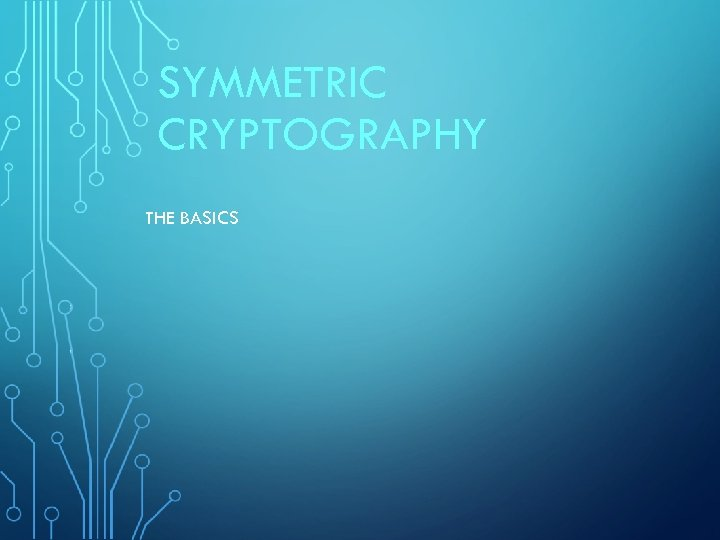SYMMETRIC CRYPTOGRAPHY THE BASICS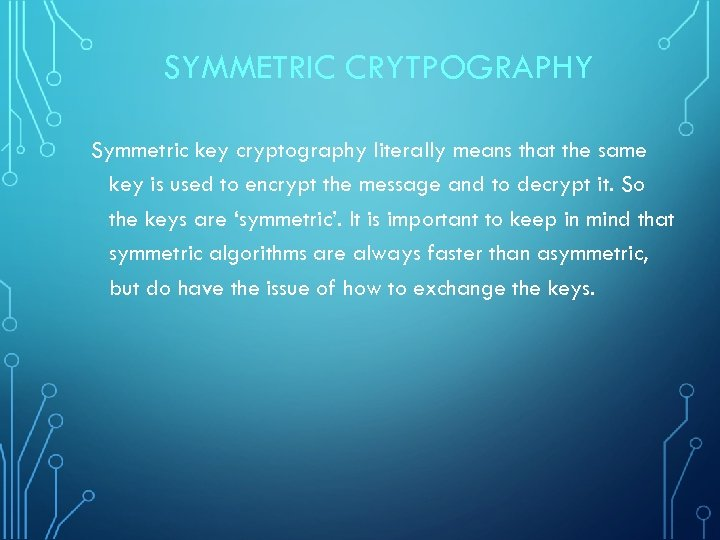SYMMETRIC CRYTPOGRAPHY Symmetric key cryptography literally means that the same key is used to encrypt the message and to decrypt it. So the keys are ‘symmetric’. It is important to keep in mind that symmetric algorithms are always faster than asymmetric, but do have the issue of how to exchange the keys.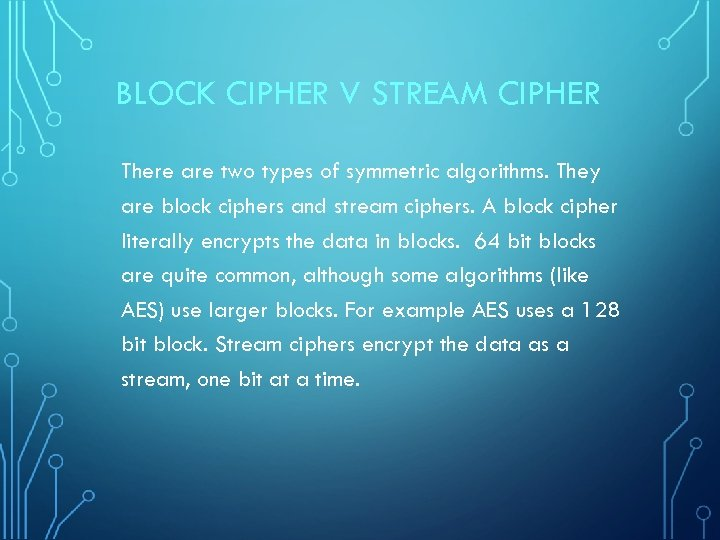BLOCK CIPHER V STREAM CIPHER There are two types of symmetric algorithms. They are block ciphers and stream ciphers. A block cipher literally encrypts the data in blocks. 64 bit blocks are quite common, although some algorithms (like AES) use larger blocks. For example AES uses a 128 bit block. Stream ciphers encrypt the data as a stream, one bit at a time.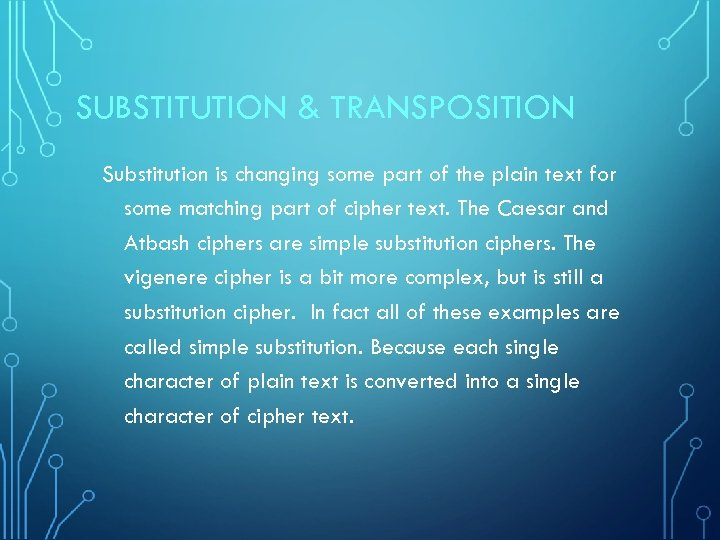SUBSTITUTION & TRANSPOSITION Substitution is changing some part of the plain text for some matching part of cipher text. The Caesar and Atbash ciphers are simple substitution ciphers. The vigenere cipher is a bit more complex, but is still a substitution cipher. In fact all of these examples are called simple substitution. Because each single character of plain text is converted into a single character of cipher text.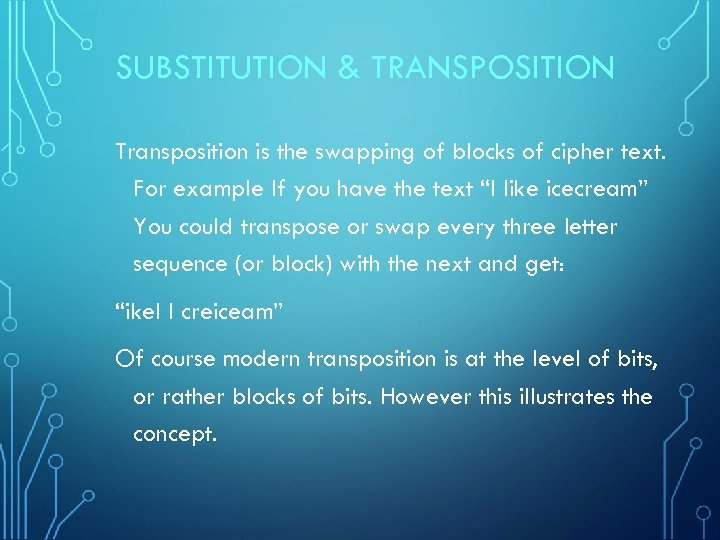SUBSTITUTION & TRANSPOSITION Transposition is the swapping of blocks of cipher text. For example If you have the text “I like icecream” You could transpose or swap every three letter sequence (or block) with the next and get: “ike. I l creiceam” Of course modern transposition is at the level of bits, or rather blocks of bits. However this illustrates the concept.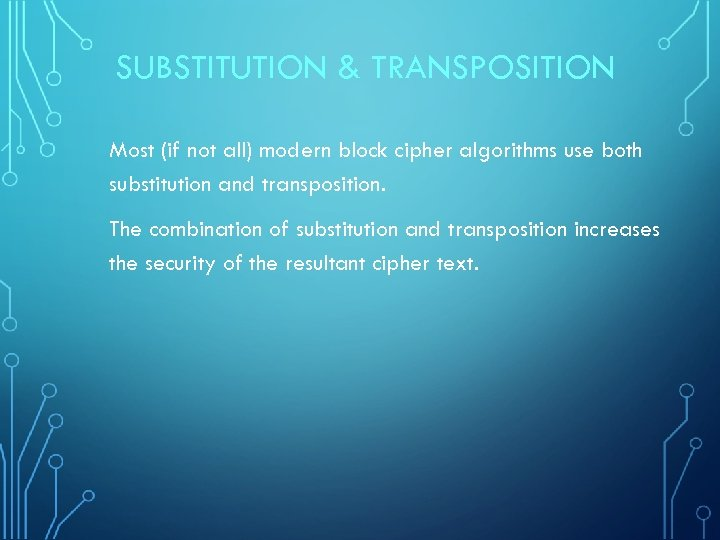SUBSTITUTION & TRANSPOSITION Most (if not all) modern block cipher algorithms use both substitution and transposition. The combination of substitution and transposition increases the security of the resultant cipher text.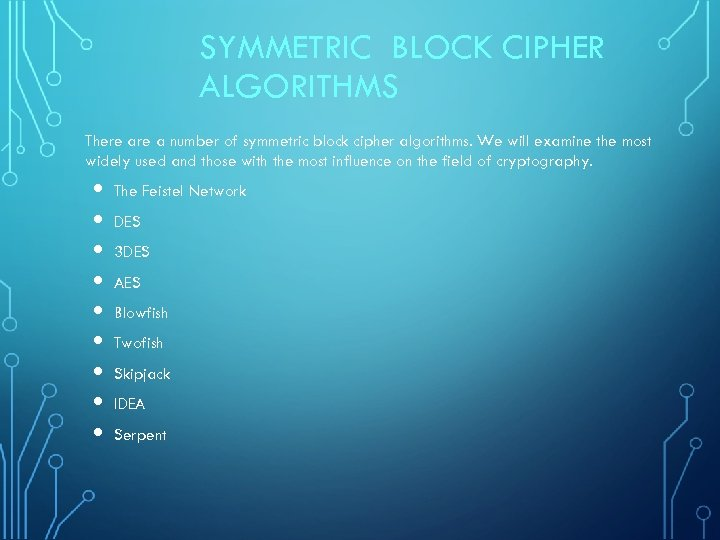SYMMETRIC BLOCK CIPHER ALGORITHMS There a number of symmetric block cipher algorithms. We will examine the most widely used and those with the most influence on the field of cryptography. The Feistel Network DES 3 DES AES Blowfish Twofish Skipjack IDEA Serpent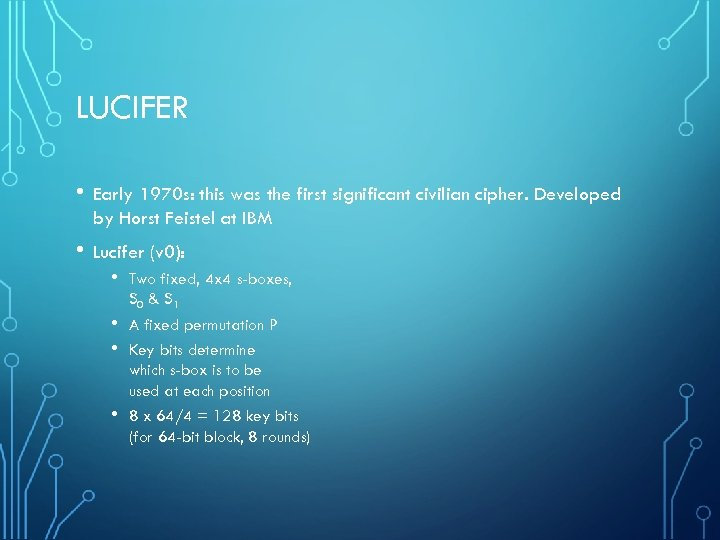LUCIFER • Early 1970 s: this was the first significant civilian cipher. Developed by Horst Feistel at IBM • Lucifer (v 0): • • Two fixed, 4 x 4 s-boxes, S 0 & S 1 A fixed permutation P Key bits determine which s-box is to be used at each position 8 x 64/4 = 128 key bits (for 64 -bit block, 8 rounds)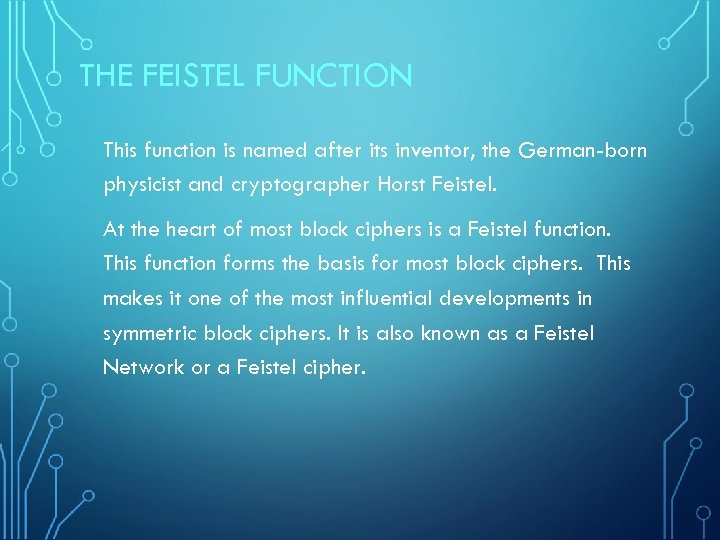THE FEISTEL FUNCTION This function is named after its inventor, the German-born physicist and cryptographer Horst Feistel. At the heart of most block ciphers is a Feistel function. This function forms the basis for most block ciphers. This makes it one of the most influential developments in symmetric block ciphers. It is also known as a Feistel Network or a Feistel cipher.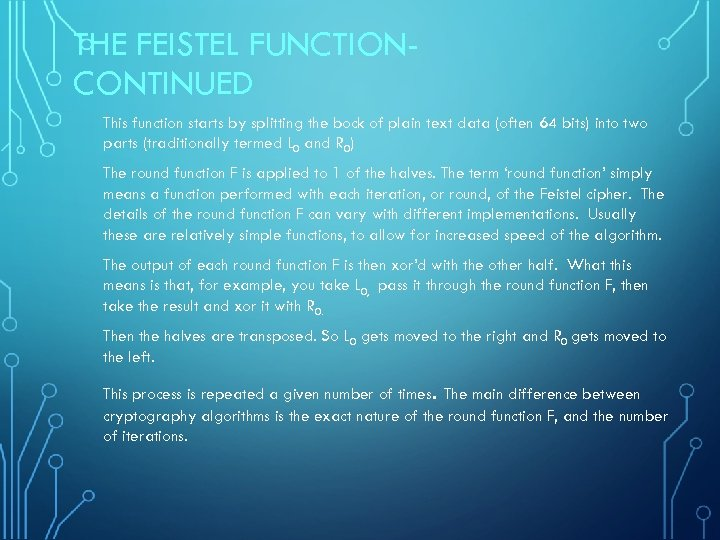THE FEISTEL FUNCTIONCONTINUED This function starts by splitting the bock of plain text data (often 64 bits) into two parts (traditionally termed L 0 and R 0) The round function F is applied to 1 of the halves. The term ‘round function’ simply means a function performed with each iteration, or round, of the Feistel cipher. The details of the round function F can vary with different implementations. Usually these are relatively simple functions, to allow for increased speed of the algorithm. The output of each round function F is then xor’d with the other half. What this means is that, for example, you take L 0, pass it through the round function F, then take the result and xor it with R 0. Then the halves are transposed. So L 0 gets moved to the right and R 0 gets moved to the left. This process is repeated a given number of times. The main difference between cryptography algorithms is the exact nature of the round function F, and the number of iterations.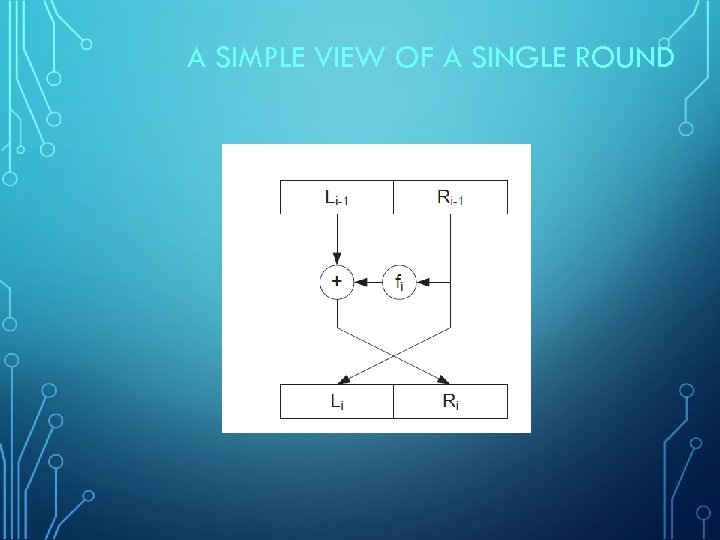A SIMPLE VIEW OF A SINGLE ROUND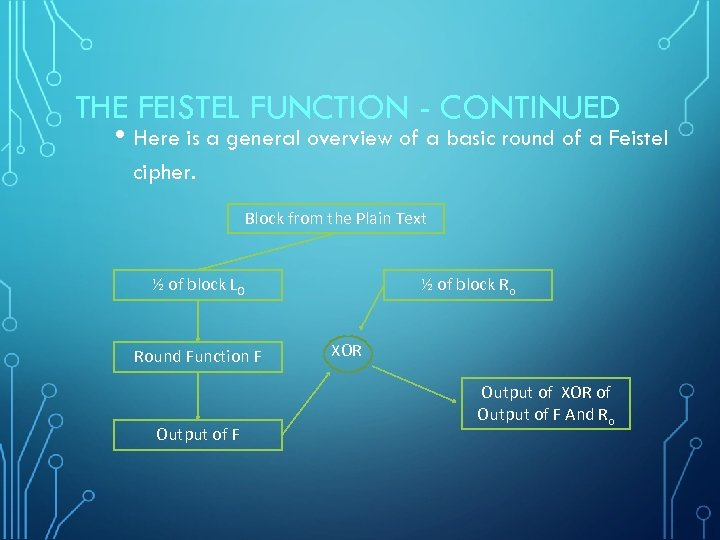THE FEISTEL FUNCTION - CONTINUED • Here is a general overview of a basic round of a Feistel cipher. Block from the Plain Text ½ of block L 0 Round Function F Output of F ½ of block Ro XOR Output of XOR of Output of F And Ro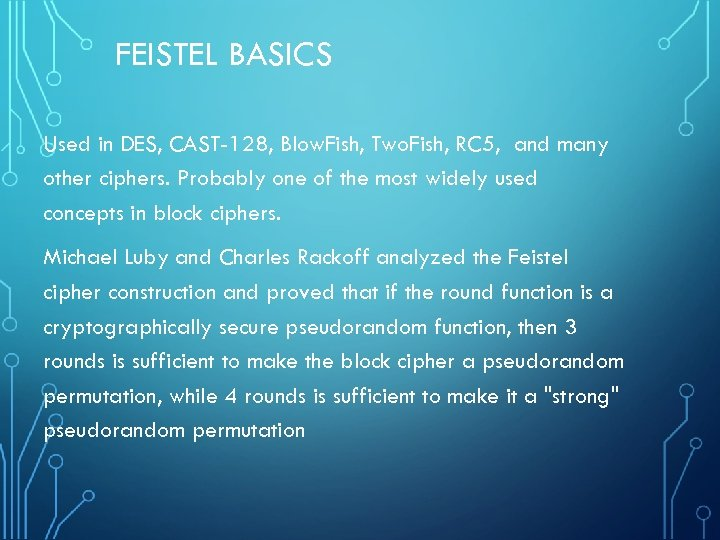FEISTEL BASICS Used in DES, CAST-128, Blow. Fish, Two. Fish, RC 5, and many other ciphers. Probably one of the most widely used concepts in block ciphers. Michael Luby and Charles Rackoff analyzed the Feistel cipher construction and proved that if the round function is a cryptographically secure pseudorandom function, then 3 rounds is sufficient to make the block cipher a pseudorandom permutation, while 4 rounds is sufficient to make it a "strong" pseudorandom permutation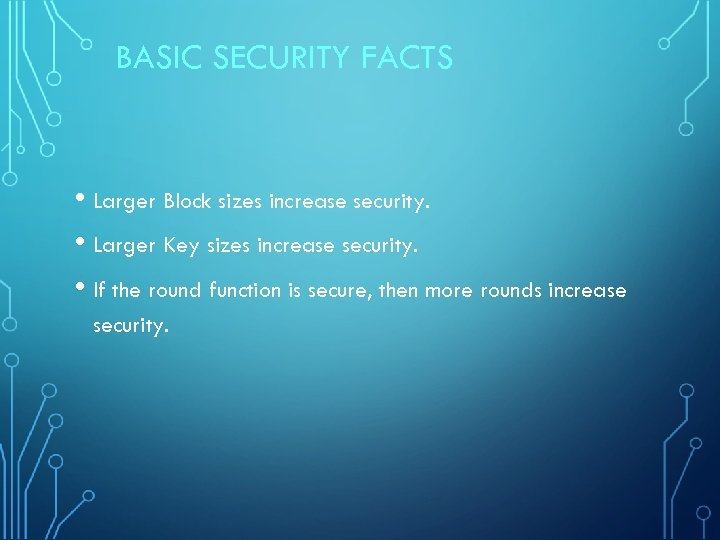BASIC SECURITY FACTS • Larger Block sizes increase security. • Larger Key sizes increase security. • If the round function is secure, then more rounds increase security.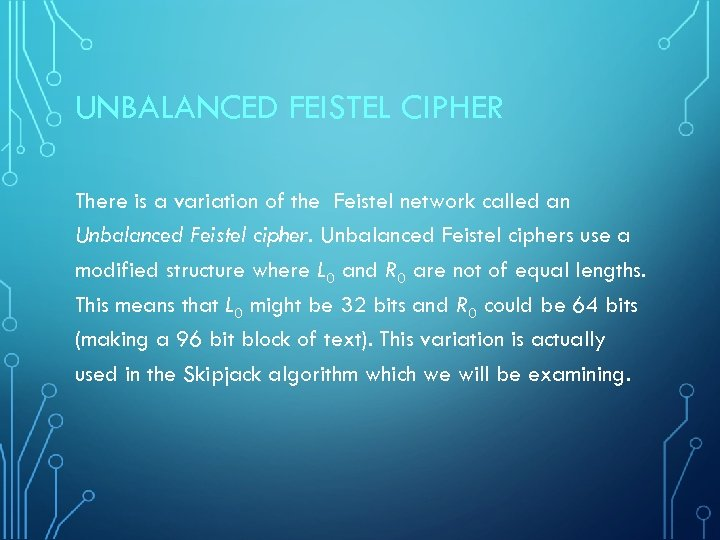UNBALANCED FEISTEL CIPHER There is a variation of the Feistel network called an Unbalanced Feistel ciphers use a modified structure where L 0 and R 0 are not of equal lengths. This means that L 0 might be 32 bits and R 0 could be 64 bits (making a 96 bit block of text). This variation is actually used in the Skipjack algorithm which we will be examining.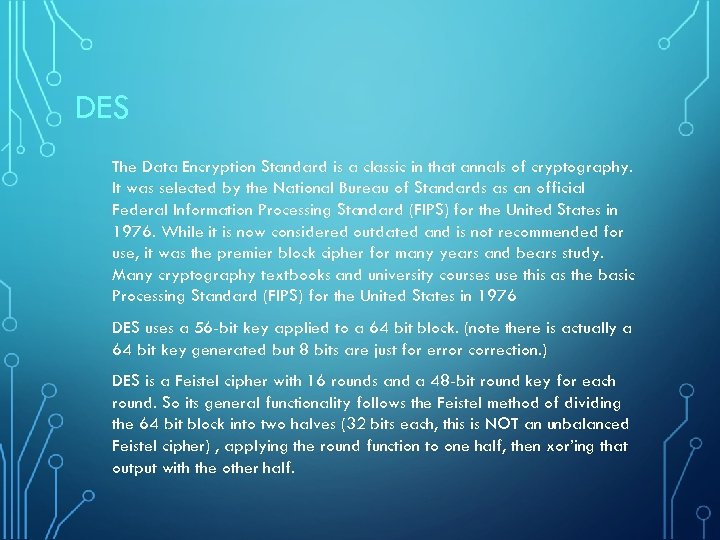DES The Data Encryption Standard is a classic in that annals of cryptography. It was selected by the National Bureau of Standards as an official Federal Information Processing Standard (FIPS) for the United States in 1976. While it is now considered outdated and is not recommended for use, it was the premier block cipher for many years and bears study. Many cryptography textbooks and university courses use this as the basic Processing Standard (FIPS) for the United States in 1976 DES uses a 56 -bit key applied to a 64 bit block. (note there is actually a 64 bit key generated but 8 bits are just for error correction. ) DES is a Feistel cipher with 16 rounds and a 48 -bit round key for each round. So its general functionality follows the Feistel method of dividing the 64 bit block into two halves (32 bits each, this is NOT an unbalanced Feistel cipher) , applying the round function to one half, then xor’ing that output with the other half.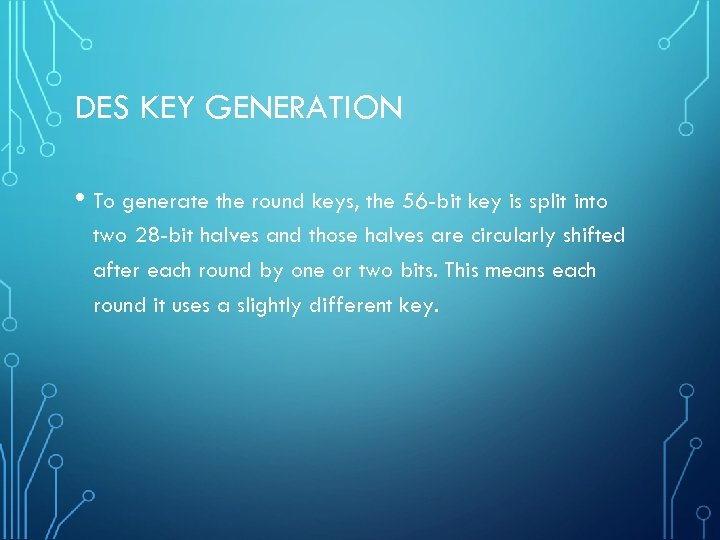DES KEY GENERATION • To generate the round keys, the 56 -bit key is split into two 28 -bit halves and those halves are circularly shifted after each round by one or two bits. This means each round it uses a slightly different key.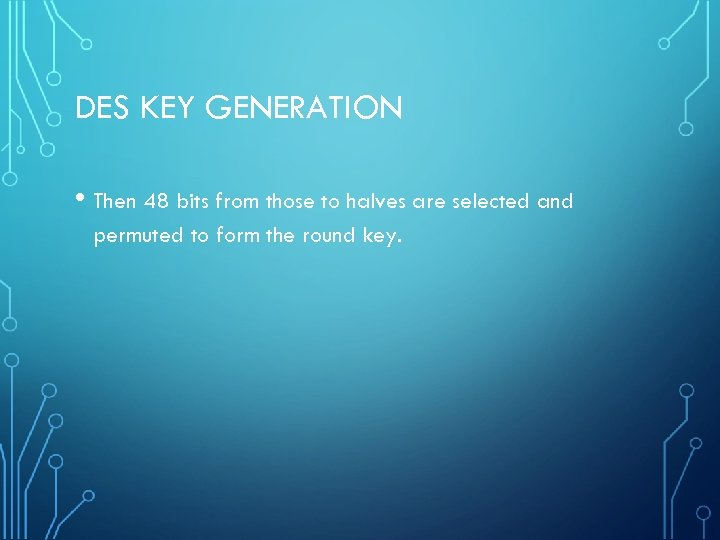DES KEY GENERATION • Then 48 bits from those to halves are selected and permuted to form the round key.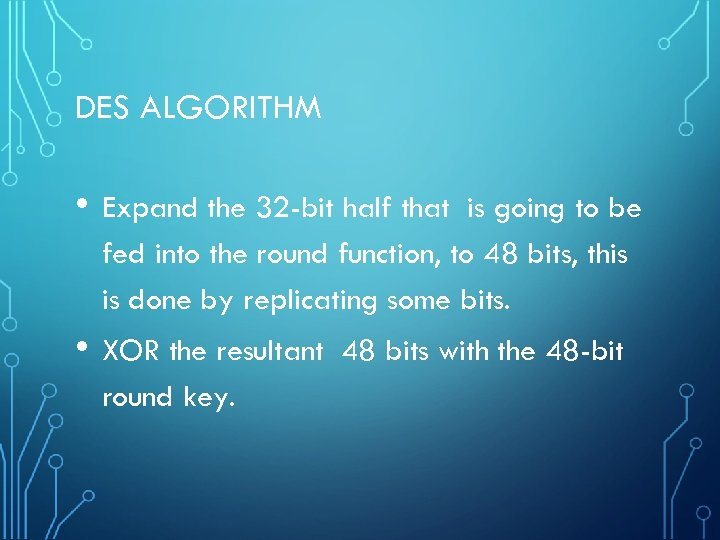DES ALGORITHM • Expand the 32 -bit half that is going to be fed into the round function, to 48 bits, this is done by replicating some bits. • XOR the resultant 48 bits with the 48 -bit round key.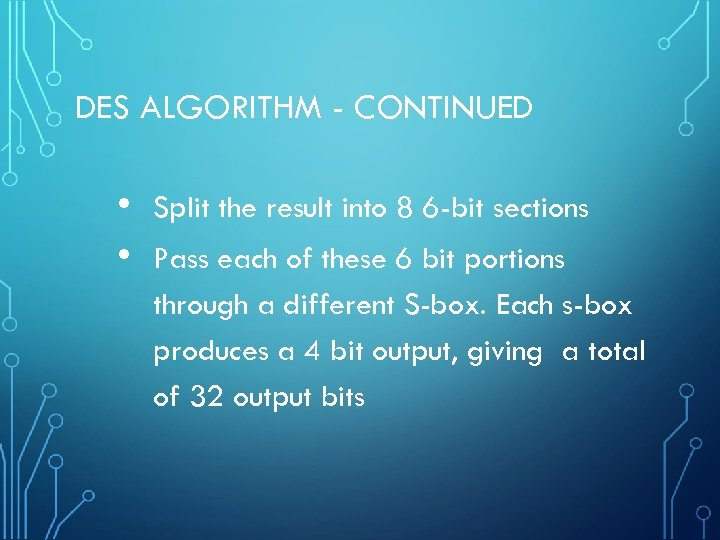DES ALGORITHM - CONTINUED • • Split the result into 8 6 -bit sections Pass each of these 6 bit portions through a different S-box. Each s-box produces a 4 bit output, giving a total of 32 output bits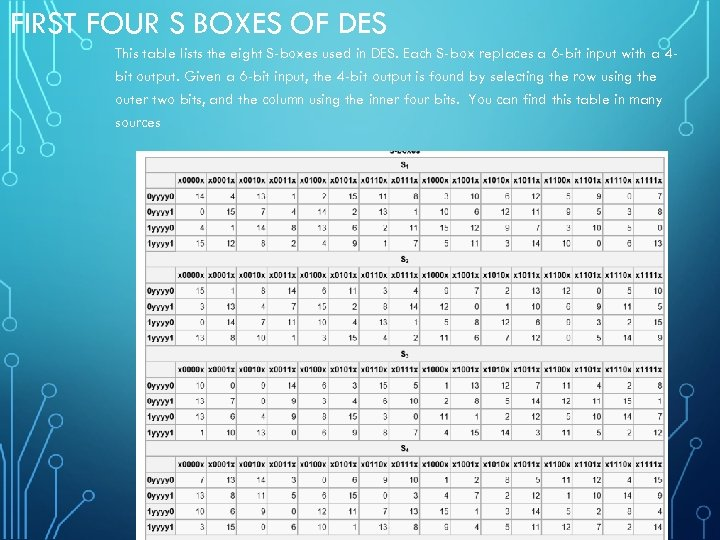FIRST FOUR S BOXES OF DES This table lists the eight S-boxes used in DES. Each S-box replaces a 6 -bit input with a 4 bit output. Given a 6 -bit input, the 4 -bit output is found by selecting the row using the outer two bits, and the column using the inner four bits. You can find this table in many sources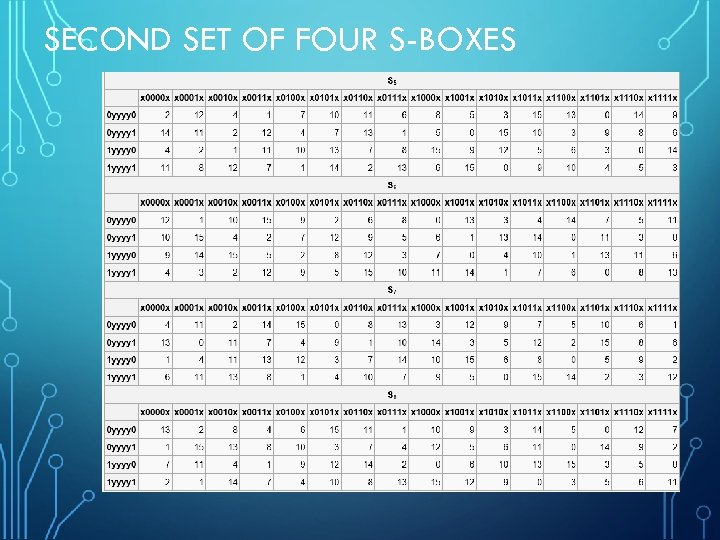SECOND SET OF FOUR S-BOXES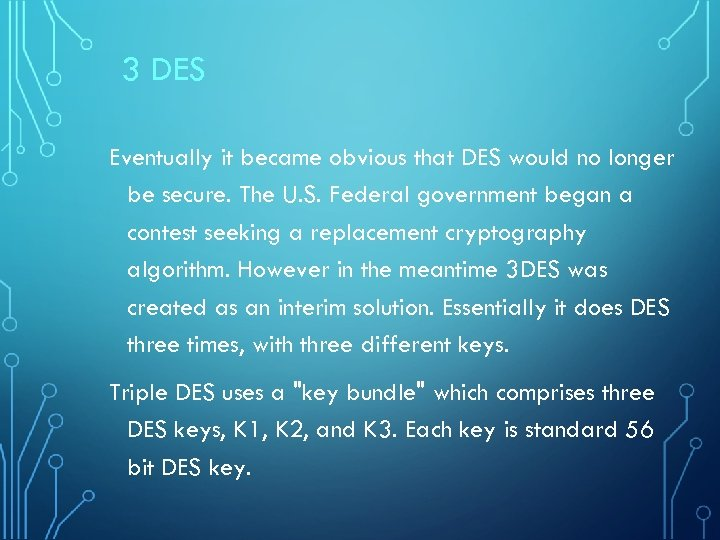3 DES Eventually it became obvious that DES would no longer be secure. The U. S. Federal government began a contest seeking a replacement cryptography algorithm. However in the meantime 3 DES was created as an interim solution. Essentially it does DES three times, with three different keys. Triple DES uses a "key bundle" which comprises three DES keys, K 1, K 2, and K 3. Each key is standard 56 bit DES key.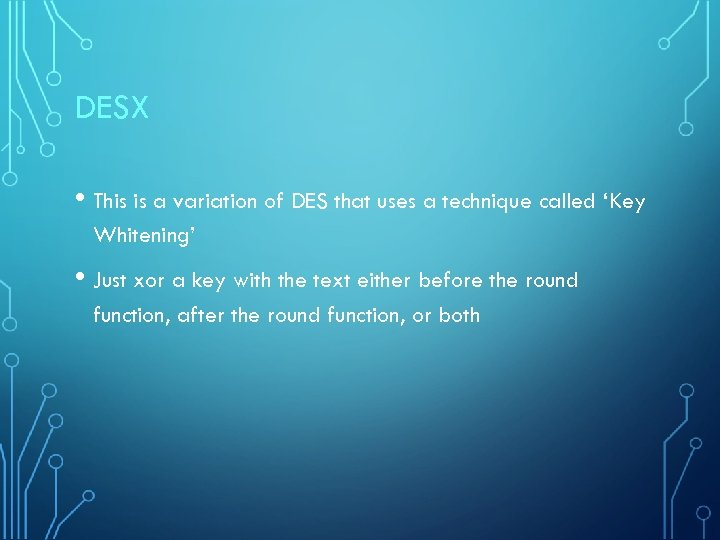DESX • This is a variation of DES that uses a technique called ‘Key Whitening’ • Just xor a key with the text either before the round function, after the round function, or both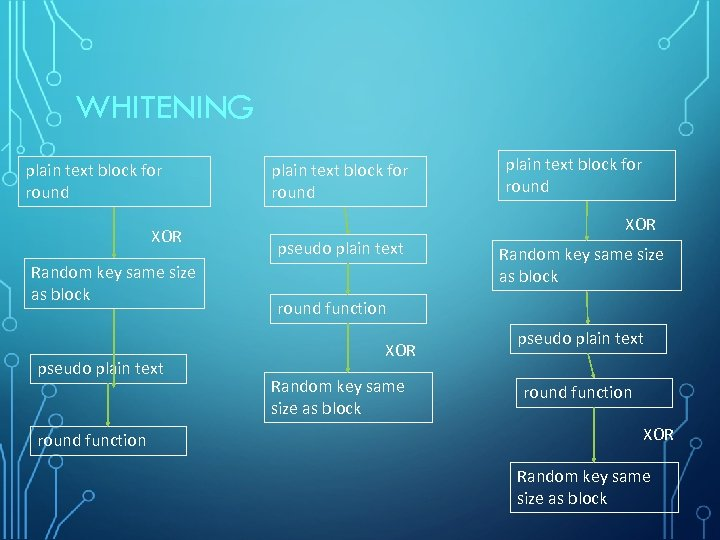WHITENING plain text block for round XOR Random key same size as block pseudo plain text round function plain text block for round XOR pseudo plain text Random key same size as block round function XOR Random key same size as block pseudo plain text round function XOR Random key same size as block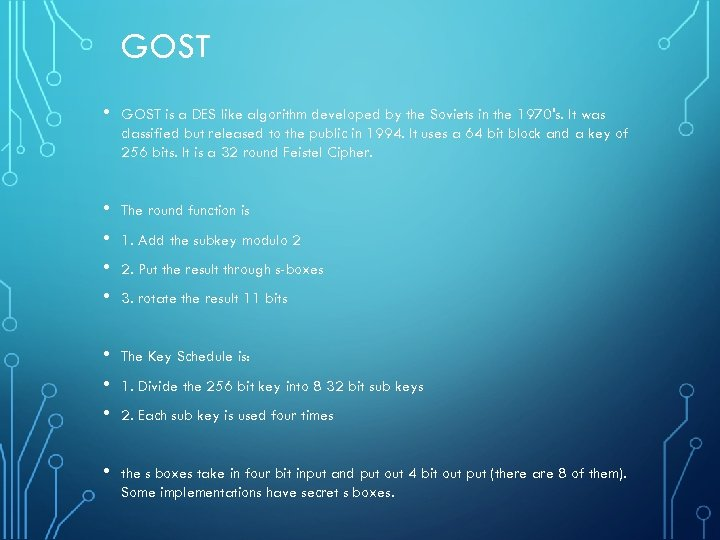GOST • GOST is a DES like algorithm developed by the Soviets in the 1970's. It was classified but released to the public in 1994. It uses a 64 bit block and a key of 256 bits. It is a 32 round Feistel Cipher. • • The round function is • • • The Key Schedule is: • the s boxes take in four bit input and put out 4 bit out put (there are 8 of them). Some implementations have secret s boxes. 1. Add the subkey modulo 2 2. Put the result through s-boxes 3. rotate the result 11 bits 1. Divide the 256 bit key into 8 32 bit sub keys 2. Each sub key is used four times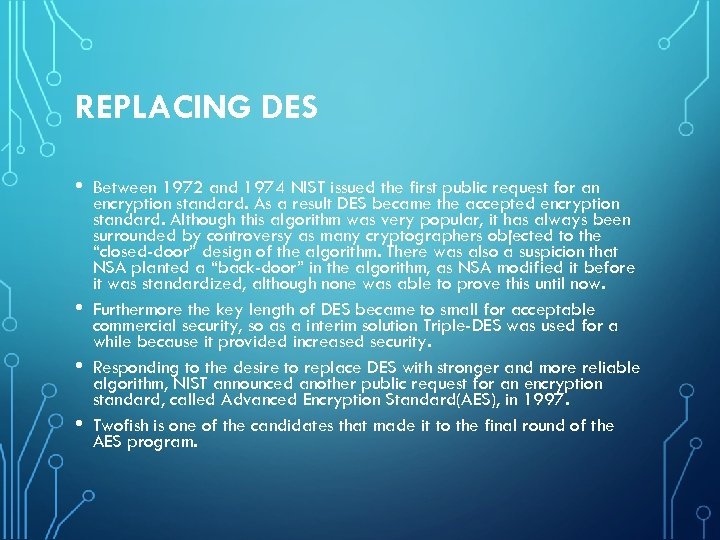REPLACING DES • • Between 1972 and 1974 NIST issued the first public request for an encryption standard. As a result DES became the accepted encryption standard. Although this algorithm was very popular, it has always been surrounded by controversy as many cryptographers objected to the “closed-door” design of the algorithm. There was also a suspicion that NSA planted a “back-door” in the algorithm, as NSA modified it before it was standardized, although none was able to prove this until now. Furthermore the key length of DES became to small for acceptable commercial security, so as a interim solution Triple-DES was used for a while because it provided increased security. Responding to the desire to replace DES with stronger and more reliable algorithm, NIST announced another public request for an encryption standard, called Advanced Encryption Standard(AES), in 1997. Twofish is one of the candidates that made it to the final round of the AES program.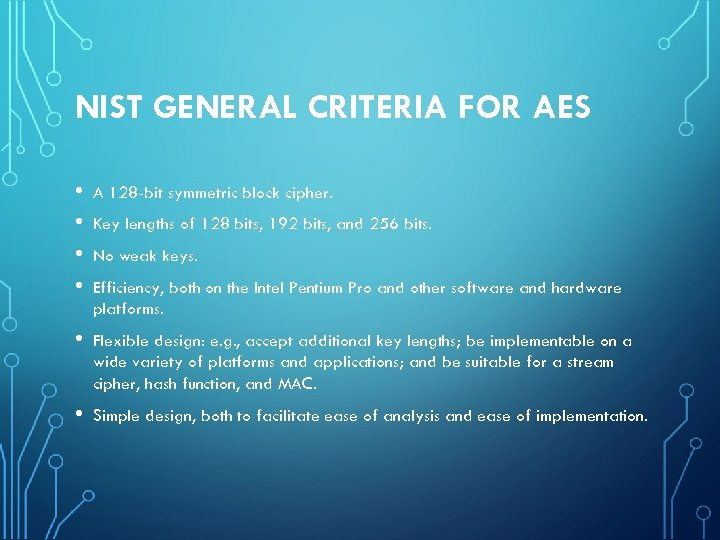NIST GENERAL CRITERIA FOR AES • • A 128 -bit symmetric block cipher. • Flexible design: e. g. , accept additional key lengths; be implementable on a wide variety of platforms and applications; and be suitable for a stream cipher, hash function, and MAC. • Simple design, both to facilitate ease of analysis and ease of implementation. Key lengths of 128 bits, 192 bits, and 256 bits. No weak keys. Efficiency, both on the Intel Pentium Pro and other software and hardware platforms.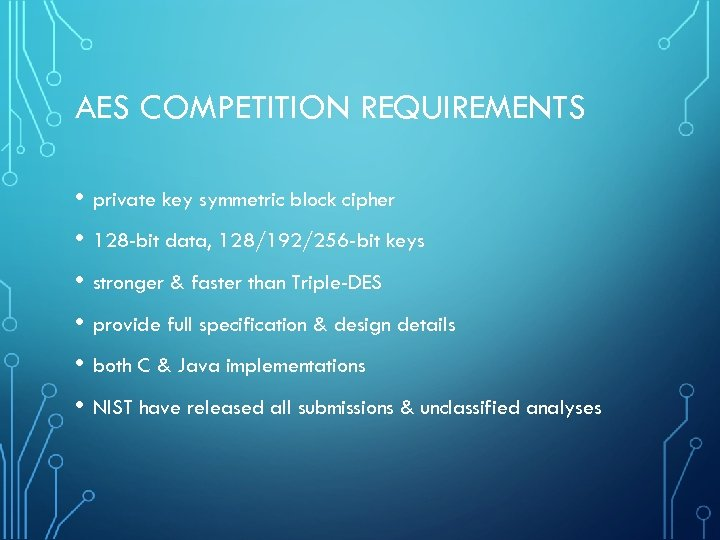AES COMPETITION REQUIREMENTS • private key symmetric block cipher • 128 -bit data, 128/192/256 -bit keys • stronger & faster than Triple-DES • provide full specification & design details • both C & Java implementations • NIST have released all submissions & unclassified analyses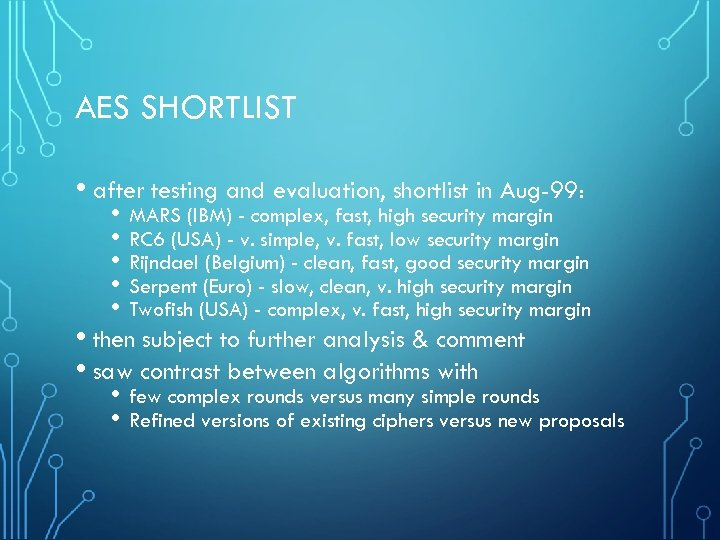AES SHORTLIST • after testing and evaluation, shortlist in Aug-99: • MARS (IBM) - complex, fast, high security margin • RC 6 (USA) - v. simple, v. fast, low security margin • Rijndael (Belgium) - clean, fast, good security margin • Serpent (Euro) - slow, clean, v. high security margin • Twofish (USA) - complex, v. fast, high security margin • then subject to further analysis & comment • saw contrast between algorithms with • few complex rounds versus many simple rounds • Refined versions of existing ciphers versus new proposals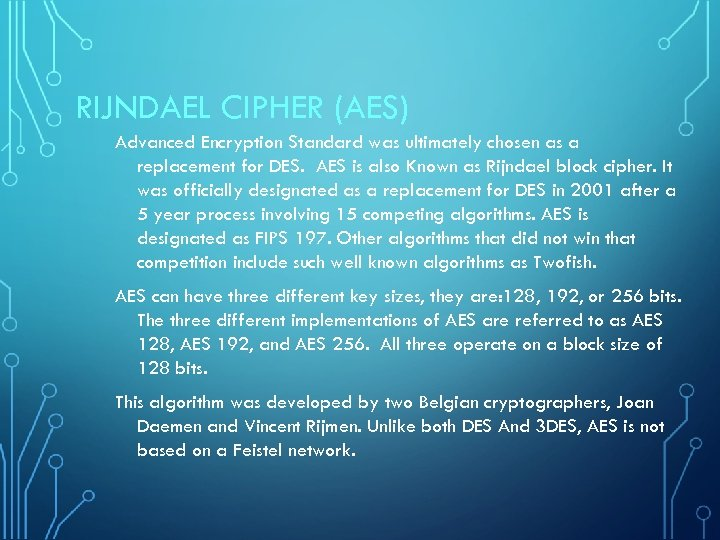RIJNDAEL CIPHER (AES) Advanced Encryption Standard was ultimately chosen as a replacement for DES. AES is also Known as Rijndael block cipher. It was officially designated as a replacement for DES in 2001 after a 5 year process involving 15 competing algorithms. AES is designated as FIPS 197. Other algorithms that did not win that competition include such well known algorithms as Twofish. AES can have three different key sizes, they are: 128, 192, or 256 bits. The three different implementations of AES are referred to as AES 128, AES 192, and AES 256. All three operate on a block size of 128 bits. This algorithm was developed by two Belgian cryptographers, Joan Daemen and Vincent Rijmen. Unlike both DES And 3 DES, AES is not based on a Feistel network.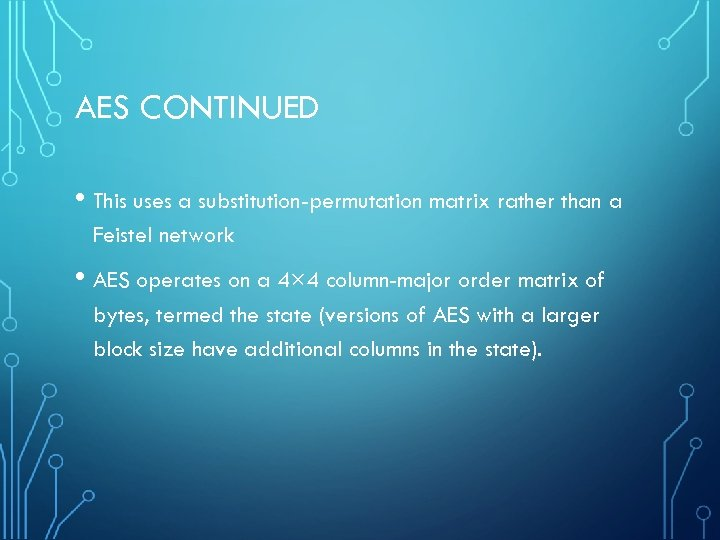AES CONTINUED • This uses a substitution-permutation matrix rather than a Feistel network • AES operates on a 4× 4 column-major order matrix of bytes, termed the state (versions of AES with a larger block size have additional columns in the state).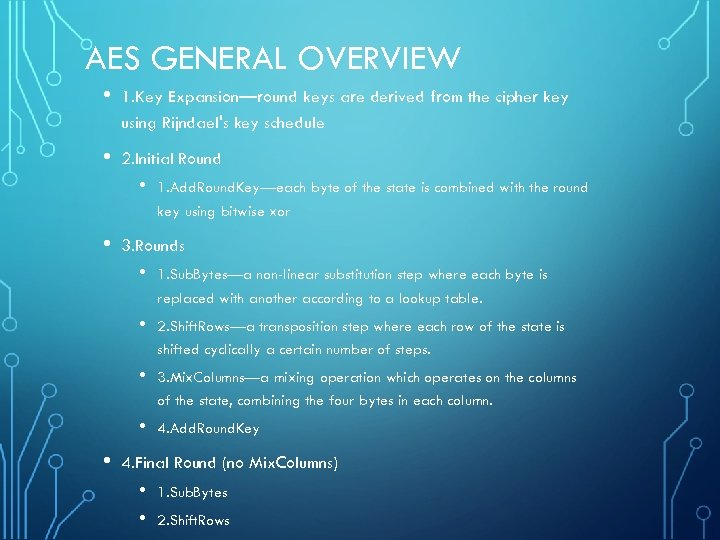AES GENERAL OVERVIEW • 1. Key Expansion—round keys are derived from the cipher key using Rijndael's key schedule • 2. Initial Round • • 1. Add. Round. Key—each byte of the state is combined with the round key using bitwise xor 3. Rounds • • 2. Shift. Rows—a transposition step where each row of the state is shifted cyclically a certain number of steps. • 3. Mix. Columns—a mixing operation which operates on the columns of the state, combining the four bytes in each column. • • 1. Sub. Bytes—a non-linear substitution step where each byte is replaced with another according to a lookup table. 4. Add. Round. Key 4. Final Round (no Mix. Columns) • • 1. Sub. Bytes 2. Shift. Rows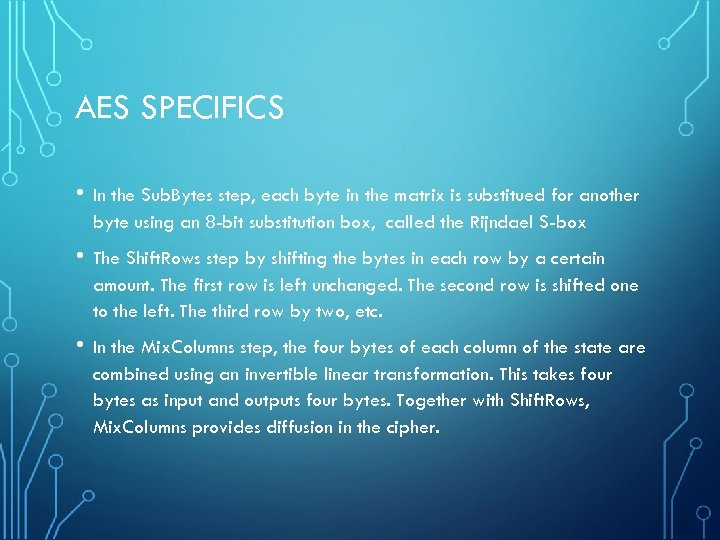AES SPECIFICS • In the Sub. Bytes step, each byte in the matrix is substitued for another byte using an 8 -bit substitution box, called the Rijndael S-box • The Shift. Rows step by shifting the bytes in each row by a certain amount. The first row is left unchanged. The second row is shifted one to the left. The third row by two, etc. • In the Mix. Columns step, the four bytes of each column of the state are combined using an invertible linear transformation. This takes four bytes as input and outputs four bytes. Together with Shift. Rows, Mix. Columns provides diffusion in the cipher.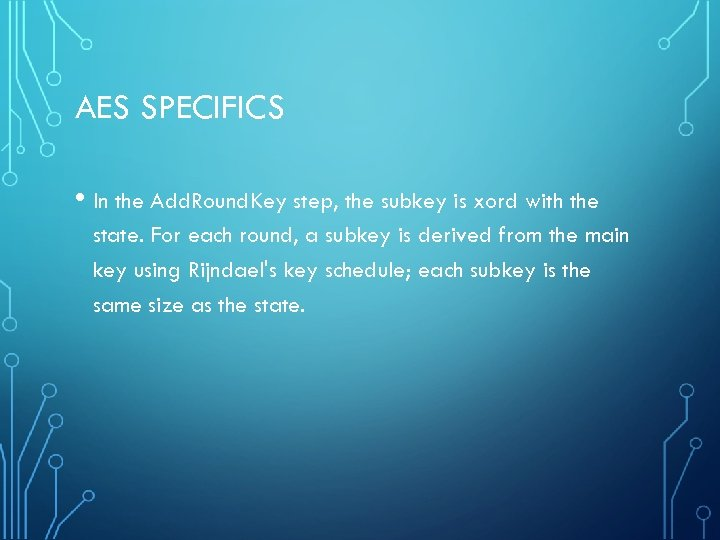AES SPECIFICS • In the Add. Round. Key step, the subkey is xord with the state. For each round, a subkey is derived from the main key using Rijndael's key schedule; each subkey is the same size as the state.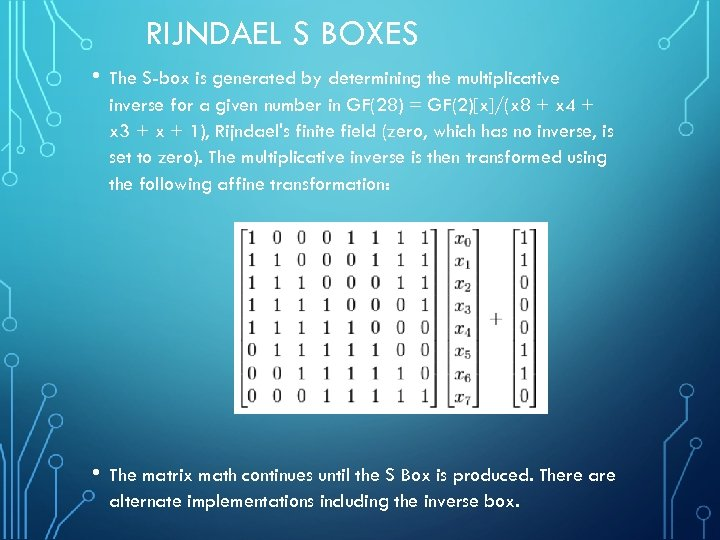RIJNDAEL S BOXES • The S-box is generated by determining the multiplicative inverse for a given number in GF(28) = GF(2)[x]/(x 8 + x 4 + x 3 + x + 1), Rijndael's finite field (zero, which has no inverse, is set to zero). The multiplicative inverse is then transformed using the following affine transformation: • The matrix math continues until the S Box is produced. There alternate implementations including the inverse box.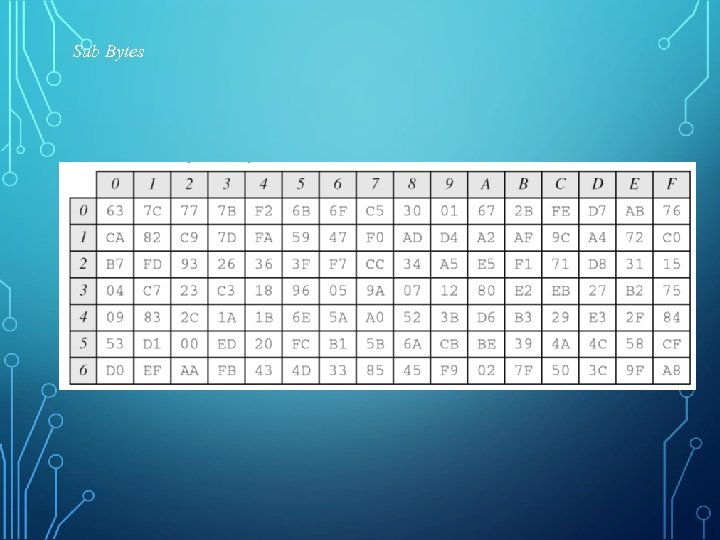Sub Bytes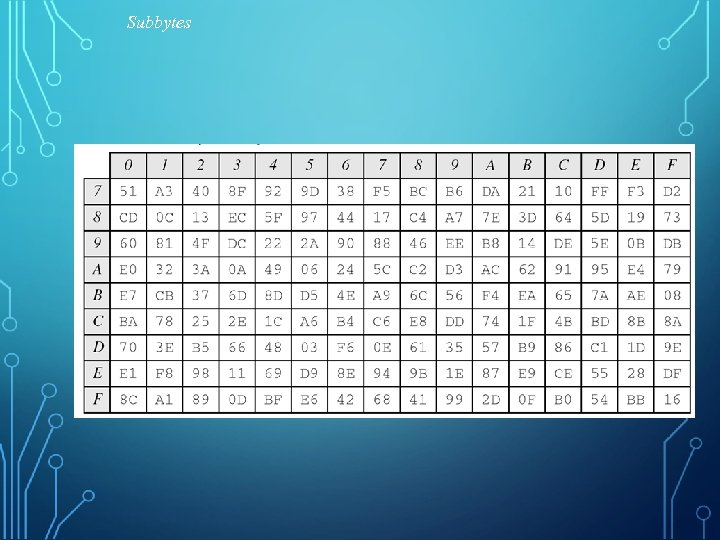Subbytes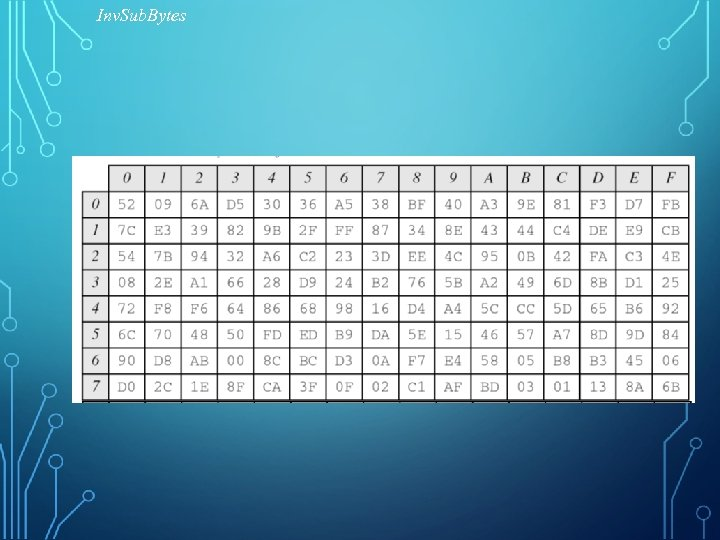Inv. Sub. Bytes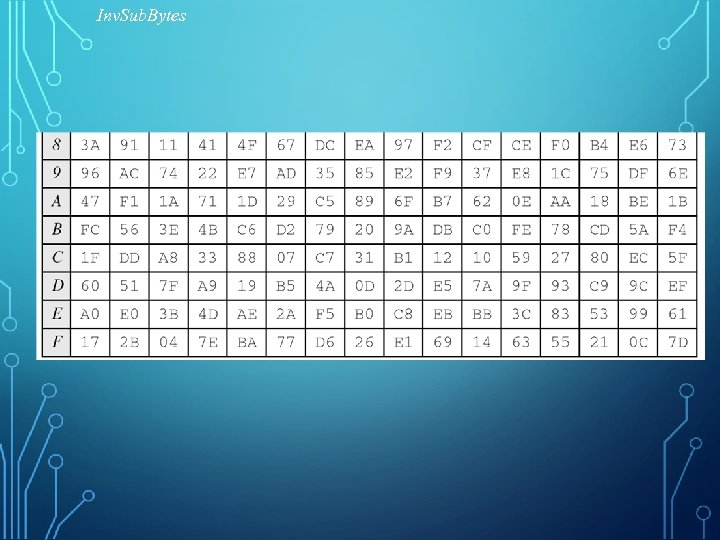Inv. Sub. Bytes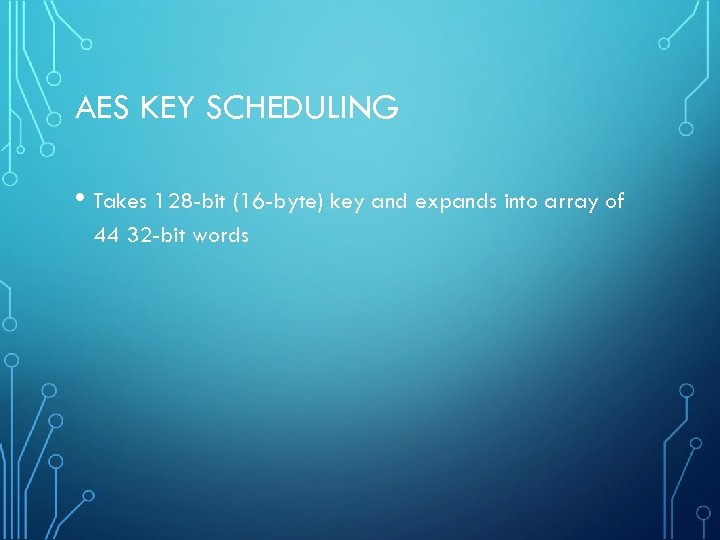AES KEY SCHEDULING • Takes 128 -bit (16 -byte) key and expands into array of 44 32 -bit words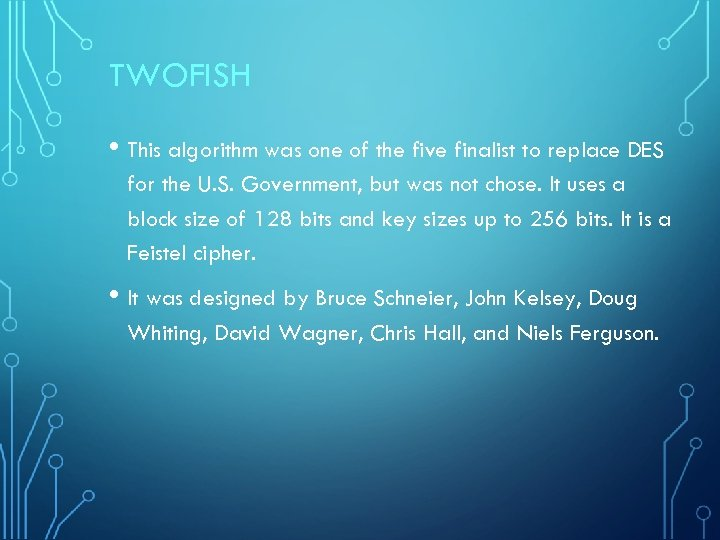TWOFISH • This algorithm was one of the five finalist to replace DES for the U. S. Government, but was not chose. It uses a block size of 128 bits and key sizes up to 256 bits. It is a Feistel cipher. • It was designed by Bruce Schneier, John Kelsey, Doug Whiting, David Wagner, Chris Hall, and Niels Ferguson.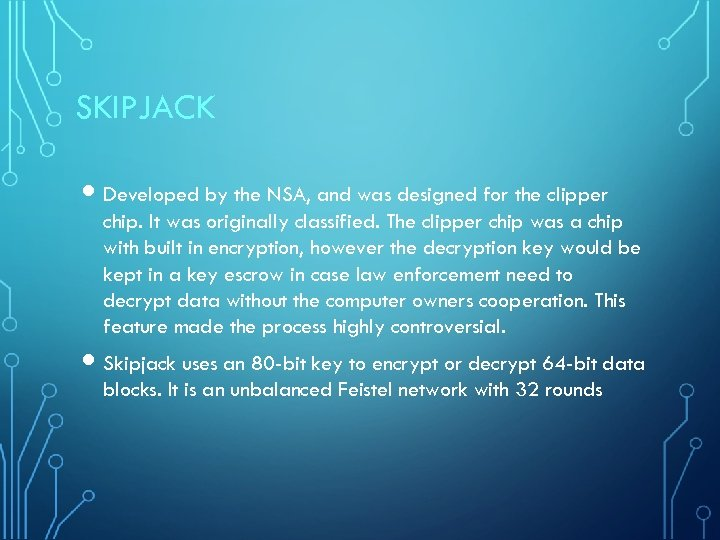SKIPJACK Developed by the NSA, and was designed for the clipper chip. It was originally classified. The clipper chip was a chip with built in encryption, however the decryption key would be kept in a key escrow in case law enforcement need to decrypt data without the computer owners cooperation. This feature made the process highly controversial. Skipjack uses an 80 -bit key to encrypt or decrypt 64 -bit data blocks. It is an unbalanced Feistel network with 32 rounds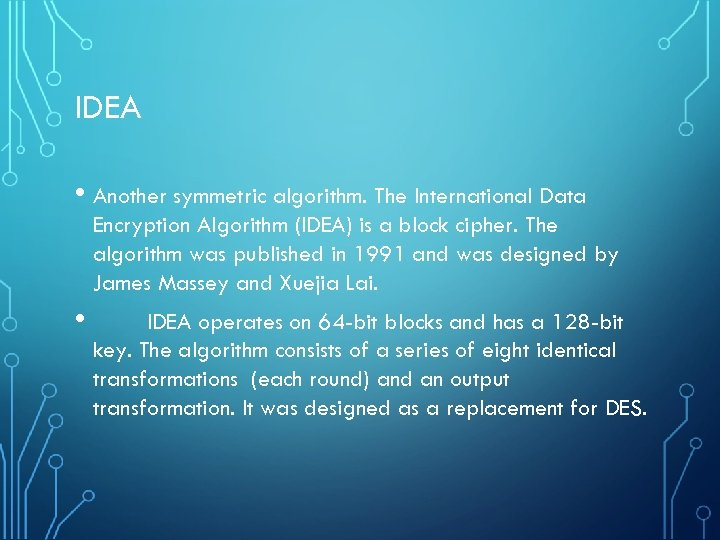IDEA • Another symmetric algorithm. The International Data Encryption Algorithm (IDEA) is a block cipher. The algorithm was published in 1991 and was designed by James Massey and Xuejia Lai. • IDEA operates on 64 -bit blocks and has a 128 -bit key. The algorithm consists of a series of eight identical transformations (each round) and an output transformation. It was designed as a replacement for DES.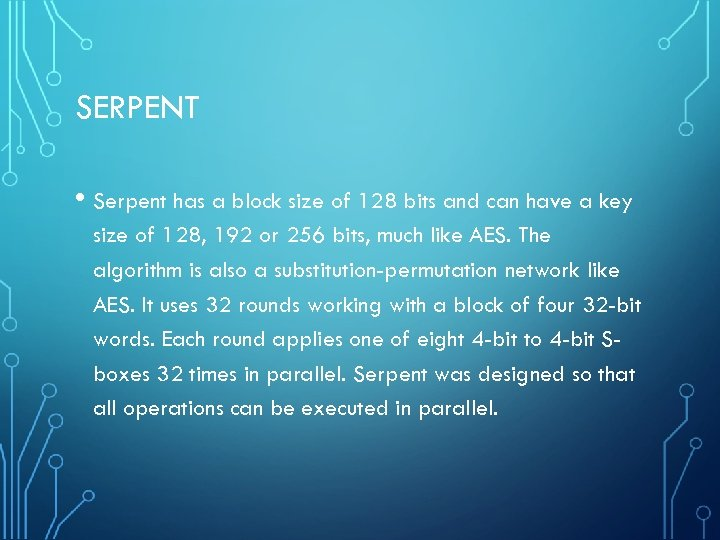SERPENT • Serpent has a block size of 128 bits and can have a key size of 128, 192 or 256 bits, much like AES. The algorithm is also a substitution-permutation network like AES. It uses 32 rounds working with a block of four 32 -bit words. Each round applies one of eight 4 -bit to 4 -bit Sboxes 32 times in parallel. Serpent was designed so that all operations can be executed in parallel.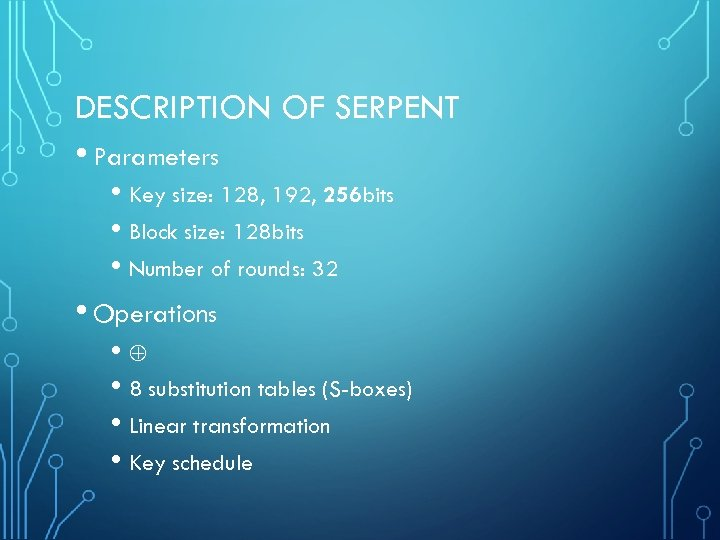DESCRIPTION OF SERPENT • Parameters • Key size: 128, 192, 256 bits • Block size: 128 bits • Number of rounds: 32 • Operations • • 8 substitution tables (S-boxes) • Linear transformation • Key schedule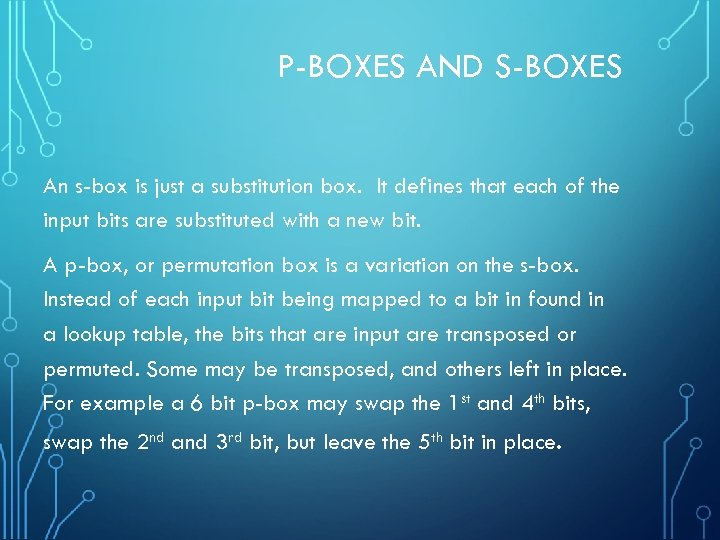P-BOXES AND S-BOXES An s-box is just a substitution box. It defines that each of the input bits are substituted with a new bit. A p-box, or permutation box is a variation on the s-box. Instead of each input bit being mapped to a bit in found in a lookup table, the bits that are input are transposed or permuted. Some may be transposed, and others left in place. For example a 6 bit p-box may swap the 1 st and 4 th bits, swap the 2 nd and 3 rd bit, but leave the 5 th bit in place.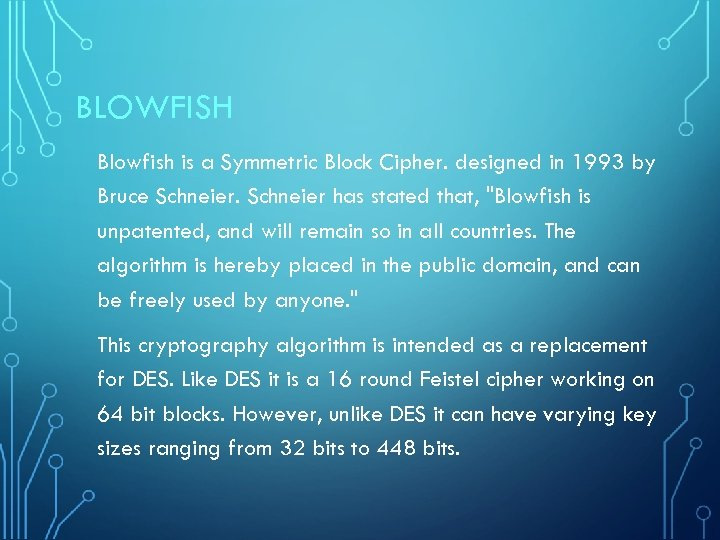BLOWFISH Blowfish is a Symmetric Block Cipher. designed in 1993 by Bruce Schneier has stated that, "Blowfish is unpatented, and will remain so in all countries. The algorithm is hereby placed in the public domain, and can be freely used by anyone. " This cryptography algorithm is intended as a replacement for DES. Like DES it is a 16 round Feistel cipher working on 64 bit blocks. However, unlike DES it can have varying key sizes ranging from 32 bits to 448 bits.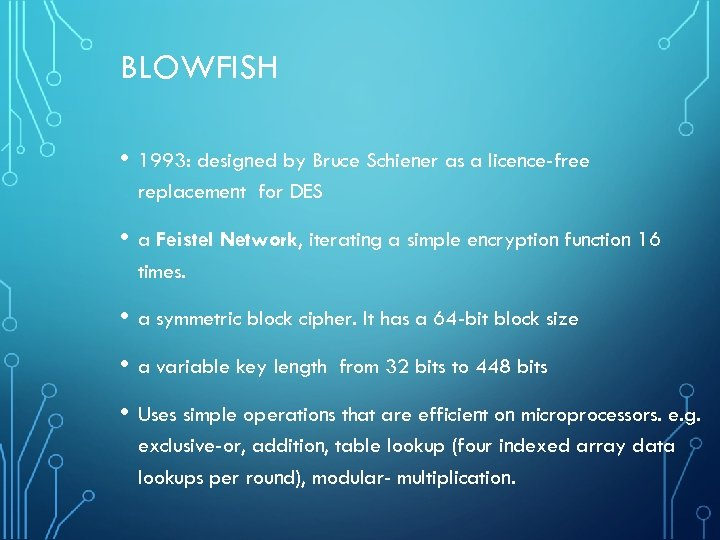BLOWFISH • 1993: designed by Bruce Schiener as a licence-free replacement for DES • a Feistel Network, iterating a simple encryption function 16 times. • a symmetric block cipher. It has a 64 -bit block size • a variable key length from 32 bits to 448 bits • Uses simple operations that are efficient on microprocessors. e. g. exclusive-or, addition, table lookup (four indexed array data lookups per round), modular- multiplication.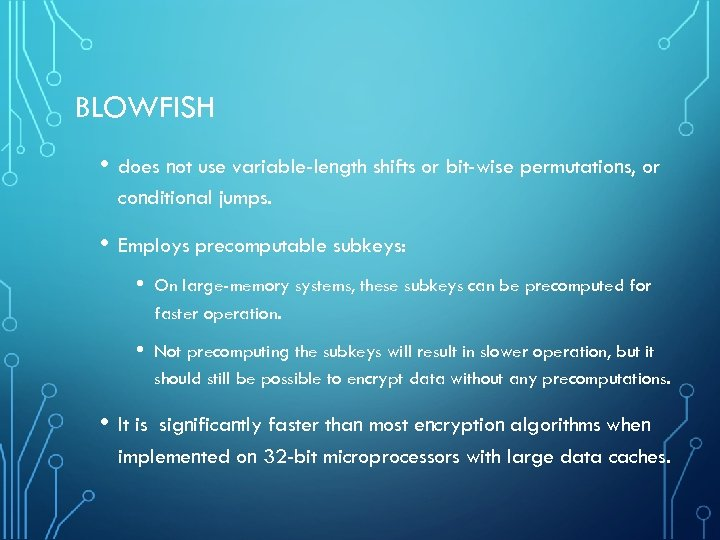BLOWFISH • does not use variable-length shifts or bit-wise permutations, or conditional jumps. • Employs precomputable subkeys: • On large-memory systems, these subkeys can be precomputed for faster operation. • Not precomputing the subkeys will result in slower operation, but it should still be possible to encrypt data without any precomputations. • It is significantly faster than most encryption algorithms when implemented on 32 -bit microprocessors with large data caches.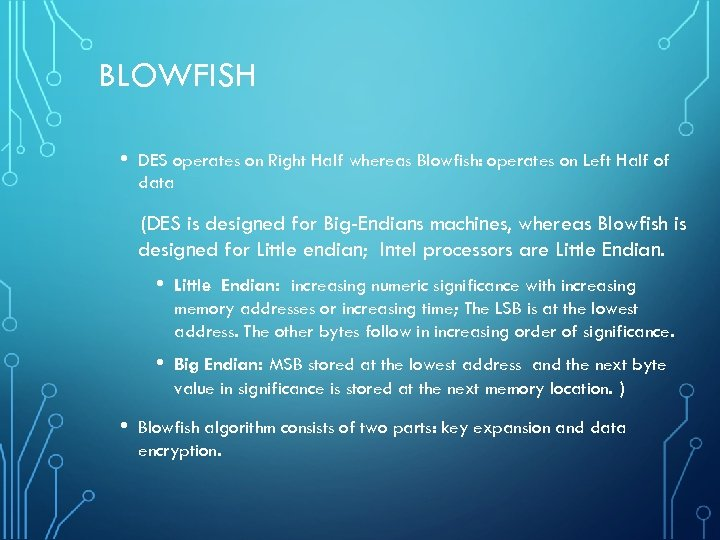BLOWFISH • DES operates on Right Half whereas Blowfish: operates on Left Half of data (DES is designed for Big-Endians machines, whereas Blowfish is designed for Little endian; Intel processors are Little Endian. • • • Little Endian: increasing numeric significance with increasing memory addresses or increasing time; The LSB is at the lowest address. The other bytes follow in increasing order of significance. Big Endian: MSB stored at the lowest address and the next byte value in significance is stored at the next memory location. ) Blowfish algorithm consists of two parts: key expansion and data encryption.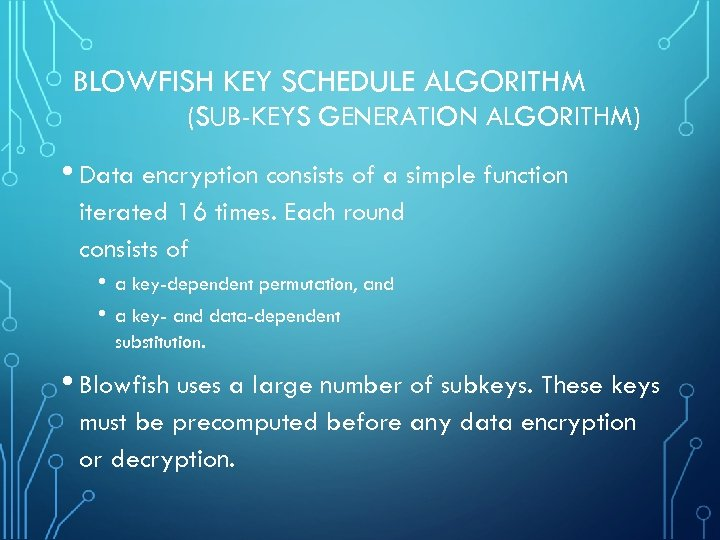BLOWFISH KEY SCHEDULE ALGORITHM (SUB-KEYS GENERATION ALGORITHM) • Data encryption consists of a simple function iterated 16 times. Each round consists of • • a key-dependent permutation, and a key- and data-dependent substitution. • Blowfish uses a large number of subkeys. These keys must be precomputed before any data encryption or decryption.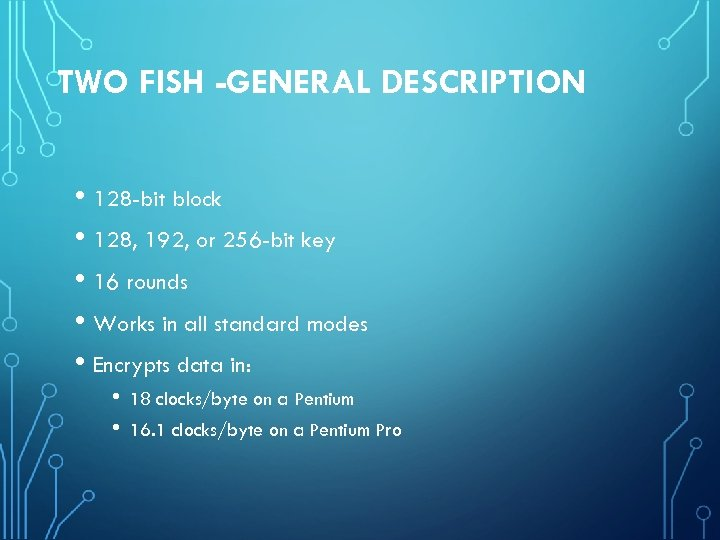TWO FISH -GENERAL DESCRIPTION • 128 -bit block • 128, 192, or 256 -bit key • 16 rounds • Works in all standard modes • Encrypts data in: • • 18 clocks/byte on a Pentium 16. 1 clocks/byte on a Pentium Pro